# Most Frequently Occurring Word

This example teaches you how to find the most frequently occurring word in Excel.

You can use the MODE function to find the most frequently occurring number. However, the MODE function only works with numbers.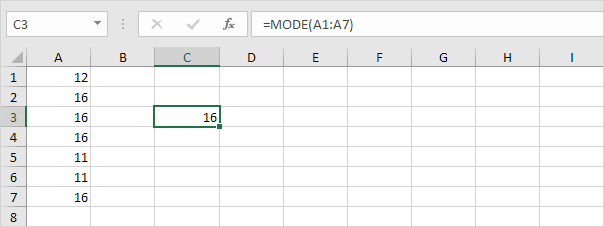You can use the COUNTIF function to count the number of occurrences of each word. However, we are looking for a single formula that returns the most frequently occurring word (circle in our example).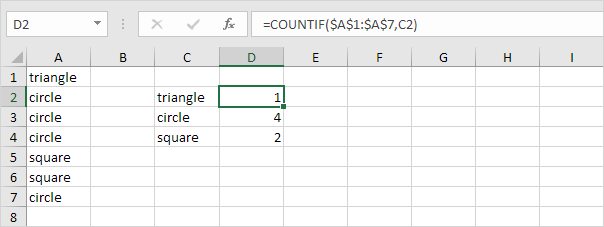To find the most frequently occurring word, execute the following steps.

1. The MATCH function returns the position of a value in a given range.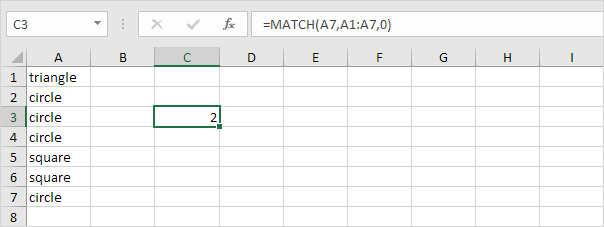Explanation: circle (A7) found at position 2 in the range A1:A7. Set the third argument to 0 to return an exact match.

2. To find the position of the most frequently occurring word (don't be overwhelmed), we add the MODE function and replace A7 with A1:A7.

3. Finish by pressing CTRL + SHIFT + ENTER.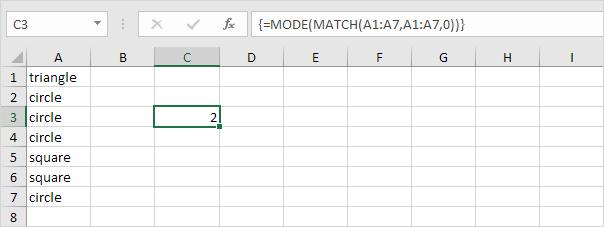Note: the formula bar indicates that this is an array formula by enclosing it in curly braces {}. Do not type these yourself. They will disappear when you edit the formula. In Excel 365 or Excel 2021, finish by simply pressing Enter. You won't see curly braces.

Explanation: the range (array constant) created by the MATCH function is stored in Excel's memory, not in a range. The array constant looks as follows:

{1;2;2;2;5;5;2} - (triangle found at position 1, circle found at position 2, circle found at position 2, etc.)

This array constant is used as an argument for the MODE function, giving a result of 2 (the position of the most frequently occurring word).

4. Use this result and the INDEX function to return the 2nd word in the range A1:A7, the most frequently occurring word.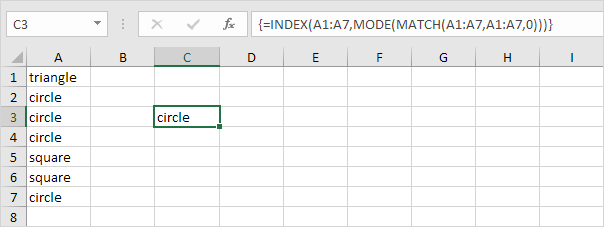Go to Next Chapter: Sort